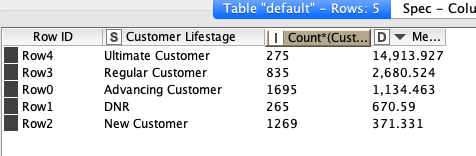# Calculating difference in values between GroupBy rows

Hi everyone,

I have the following GroupBy output:What I would like to do is to calculate the difference in the values to the right between each row in descending order so that the output would be:

Row ID Difference
Row3 12 233 (14913-2680)
Row0 1 546 (2680-1134)
etc…

That would be the best way of doing this?

Thanks for the help.

1 Like

Good question. There is the Moving Aggregation node that can give the difference by using the “range” option, but then you loose the sign of the difference (pos or neg). The other option is to create Lists (pairs) with the Moving Aggregation node, then Split Collection Column, and then calculate the difference between the columns with the Math node. But it would be nice if there would be a more elegant option.

1 Like

Hi @cason,

Did you take a look at the Lag Column node (https://www.knime.com/blog/all-you-need-is-the-lag-column-node)? You can use it to create a new, “shifted”, column and use the Math Formula afterwards to do the subtraction.

Cheers,
Simon

3 Likes

This topic was automatically closed 7 days after the last reply. New replies are no longer allowed.